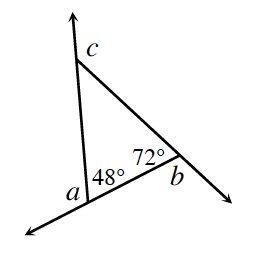Home > CCG > Chapter Ch7 > Lesson 7.2.5 > Problem7-98

7-98.$∠a$, $∠b$, and $∠c$ are exterior angles of the triangle at right. Find $m∠a$, $m∠b$, and $m∠c$. Then find $m∠a+m∠b+m∠c$.

How do the angles within a triangle relate to each other? How do the exterior angles relate to those within the triangle?